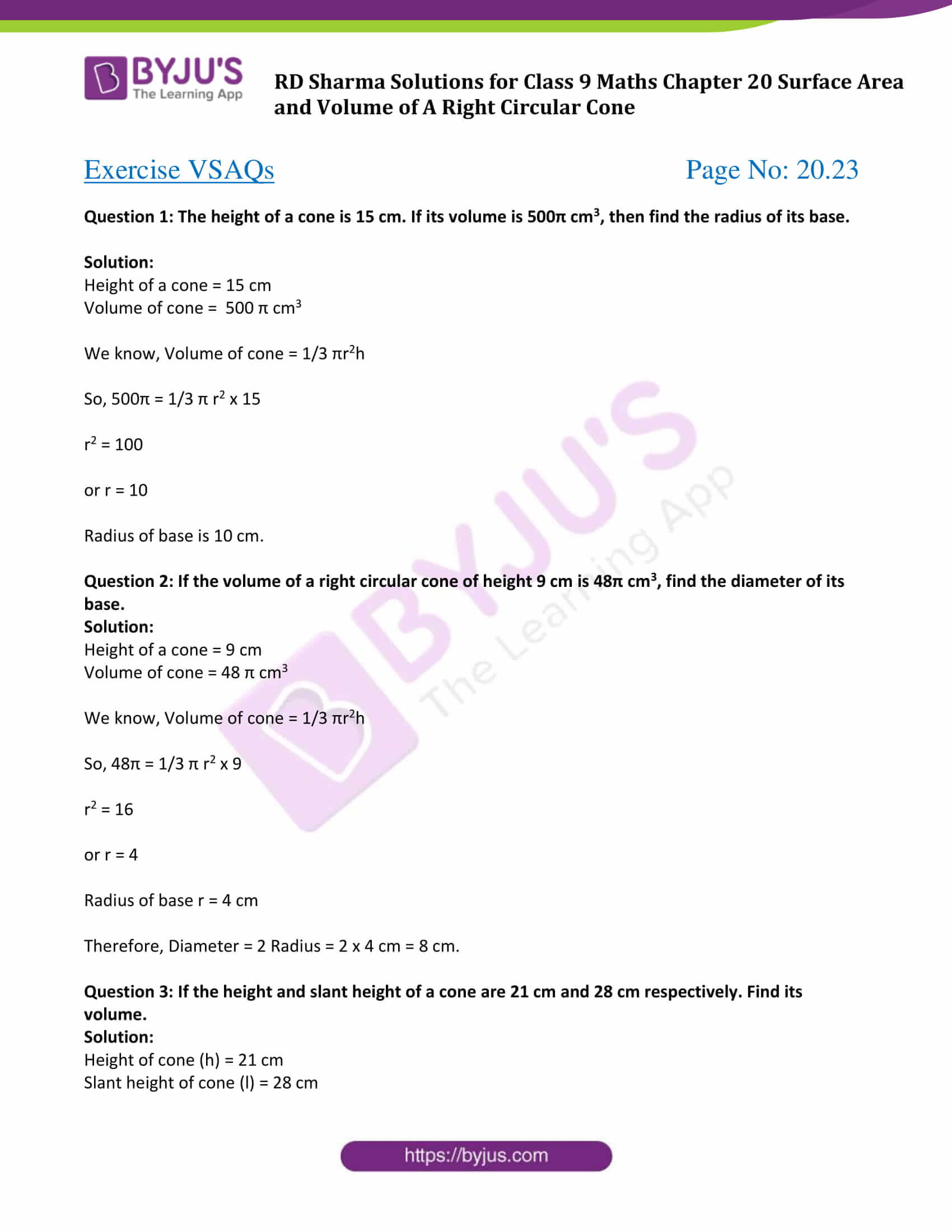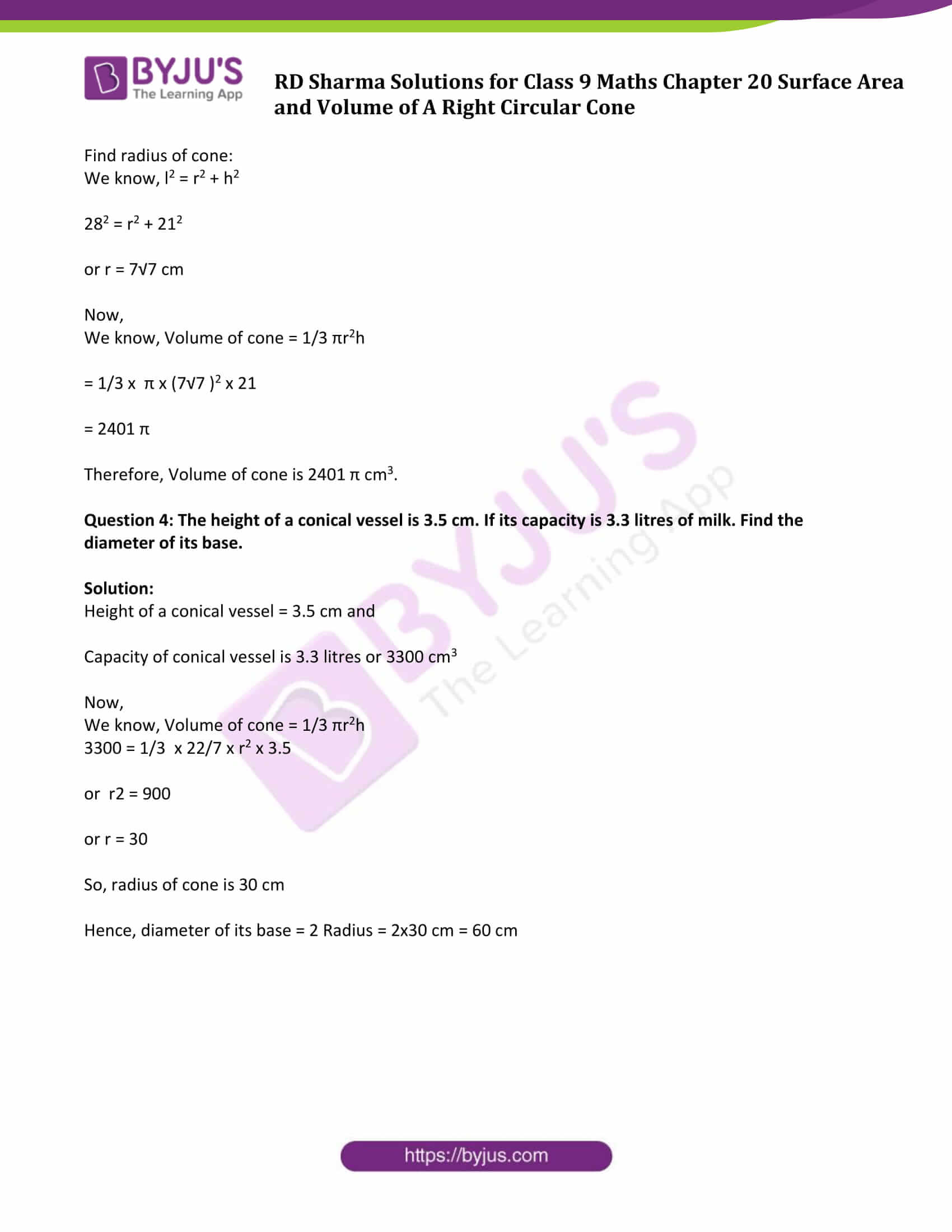# RD Sharma Solutions for Class 9 Maths Chapter 20 Surface Area and Volume of A Right Circular Cone Exercise VSAQs

This exercise solutions will help students understand the chapter in a much better way. After practicing RD Sharma solutions students will be able to solve easily any complex question based on right circular cone. These solutions will also help students understand the concepts in depth and develop a strong base for further studies. Students can get their Exercise VSAQs copy by clicking on the link below.

## Download PDF of RD Sharma Solutions for Class 9 Maths Chapter 20 Surface Area and Volume of A Right Circular Cone Exercise VSAQs### Access Answers to Maths RD Sharma Solutions for Class 9 Chapter 20 Surface Area and Volume of A Right Circular Cone Exercise VSAQs Page number 20.23

Question 1: The height of a cone is 15 cm. If its volume is 500π cm3, then find the radius of its base.

Solution:

Height of a cone = 15 cm

Volume of cone = 500 π cm3

We know, Volume of cone = 1/3 πr2h

So, 500π = 1/3 π r2 x 15

r2 = 100

or r = 10

Radius of base is 10 cm.

Question 2: If the volume of a right circular cone of height 9 cm is 48π cm3, find the diameter of its base.

Solution:

Height of a cone = 9 cm

Volume of cone = 48 π cm3

We know, Volume of cone = 1/3 πr2h

So, 48π = 1/3 π r2 x 9

r2 = 16

or r = 4

Radius of base r = 4 cm

Therefore, Diameter = 2 Radius = 2 x 4 cm = 8 cm.

Question 3: If the height and slant height of a cone are 21 cm and 28 cm respectively. Find its volume.

Solution:

Height of cone (h) = 21 cm

Slant height of cone (l) = 28 cm

We know, l2 = r2 + h2

282 = r2 + 212

or r = 7√7 cm

Now,

We know, Volume of cone = 1/3 πr2h

= 1/3 x π x (7√7 )2 x 21

= 2401 π

Therefore, Volume of cone is 2401 π cm3.

Question 4: The height of a conical vessel is 3.5 cm. If its capacity is 3.3 litres of milk. Find the diameter of its base.

Solution:

Height of a conical vessel = 3.5 cm and

Capacity of conical vessel is 3.3 litres or 3300 cm3

Now,

We know, Volume of cone = 1/3 πr2h

3300 = 1/3 x 22/7 x r2 x 3.5

or r2 = 900

or r = 30

So, radius of cone is 30 cm

Hence, diameter of its base = 2 Radius = 2×30 cm = 60 cm

## RD Sharma Solutions for Class 9 Maths Chapter 20 Surface Area and Volume of A Right Circular Cone Exercise VSAQs

RD Sharma Solutions Class 9 Maths Chapter 20 Surface Area and Volume of A Right Circular Cone Exercise VSAQs is based on the topic – Volume and surface area of a Right Circular Cone. In this exercise, students will practice problems on the volume and surface area of a right circular cone. The formulae of the volume and surface area of a right circular cone are very useful in every-day life, since we come across conical figures almost every step.